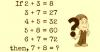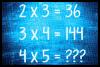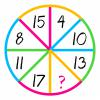BRAIN TEASERSBrain Teasers User Profile

# Sefa Memiş

rank
285
points
12
See full ranking list
short ranking list
 283 Đinh Hương Thịnh 12 284 William Lamb 12 285 Sefa Memiş 12 286 Devon Bishop Volare 11 287 Chan Alan 11
 Solve Math Puzzle If 2 + 3 = 8, 3 + 7 = 27, 4 + 5 = 32, 5 + 8 = 60, 6 + 7 = 72, then, 7 + 8 = ?How much is 4x5? How much is 4x5?MATH PUZZLE: Can you replace... MATH PUZZLE: Can you replace the question mark with a number?